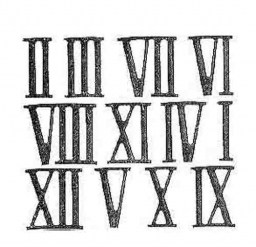# Different 44621

Express the number 68 as this property's sum of two different summands. If you divide a larger sum by a smaller sum, will you receive a result of 3?

a =  51
b =  17

### Step-by-step explanation:

a+b=68
a=3b

a+b=68
a=3·b

a+b = 68
a-3b = 0

Row 2 - Row 1 → Row 2
a+b = 68
-4b = -68

b = -68/-4 = 17
a = 68-b = 68-17 = 51

a = 51
b = 17

Our linear equations calculator calculates it.Did you find an error or inaccuracy? Feel free to write us. Thank you!

Tips for related online calculators
Do you have a system of equations and looking for calculator system of linear equations?
Do you want to perform natural numbers division - find the quotient and remainder?